JMSLTM Numerical Library 7.2.0
com.imsl.stat

## Class SignTest

• All Implemented Interfaces:
Serializable, Cloneable

```public class SignTest
extends Object
implements Serializable, Cloneable```
Performs a sign test.

Class `SignTest` tests hypotheses about the proportion p of a population that lies below a value q, where p corresponds to `percentage` and q corresponds to `percentile` in the `setPercentage` and `setPercentile` methods, respectively. In continuous distributions, this can be a test that q is the 100 p-th percentile of the population from which `x` was obtained. To carry out testing, `SignTest` tallies the number of values above q in the number of positive differences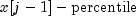for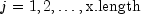. The binomial probability of the number of values above q in the number of positive differencesfor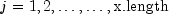or more values above q is then computed using the proportion p and the sample size in `x` (adjusted for the missing observations and ties).

Hypothesis testing is performed as follows for the usual null and alternative hypotheses:

•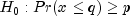(the p-th quantile is at least q)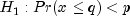Reject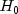if probability is less than or equal to the significance level
•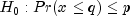(the p-th quantile is at least q)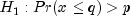Rejectif probability is greater than or equal to 1 minus the significance level
•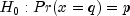(the p-th quantile is q)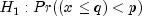or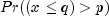Rejectif probability is less than or equal to half the significance level or greater than or equal to 1 minus half the significance level

The assumptions are as follows:

1. They are independent and identically distributed.
2. Measurement scale is at least ordinal; i.e., an ordering less than, greater than, and equal to exists in the observations.

Many uses for the sign test are possible with various values of p and q. For example, to perform a matched sample test that the difference of the medians of y and z is 0.0, let p = 0.5, q = 0.0, and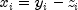in matched observations y and z. To test that the median difference is c, let q = c.

Example 1, Example 2, Serialized Form
• ### Constructor Summary

Constructors
Constructor and Description
`SignTest(double[] x)`
Constructor for `SignTest`.
• ### Method Summary

Methods
Modifier and Type Method and Description
`double` `compute()`
Performs a sign test.
`int` `getNumPositiveDev()`
Returns the number of positive differences.
`int` `getNumZeroDev()`
Returns the number of zero differences.
`void` `setPercentage(double percentage)`
Sets the percentage percentile of the population.
`void` `setPercentile(double percentile)`
Sets the hypothesized percentile of the population.
• ### Methods inherited from class java.lang.Object

`clone, equals, finalize, getClass, hashCode, notify, notifyAll, toString, wait, wait, wait`
• ### Constructor Detail

• #### SignTest

`public SignTest(double[] x)`
Constructor for `SignTest`.
Parameters:
`x` - A `double` array containing the data.
• ### Method Detail

• #### compute

`public final double compute()`
Performs a sign test.
Returns:
A `double` scalar containing the Binomial probability of `getNumPositiveDev` or more positive differences in `x.length` - number of zero differences trials. Call this value probability. If using default values, the null hypothesis is that the median equals 0.0.
• #### getNumPositiveDev

`public int getNumPositiveDev()`
Returns the number of positive differences. Note that the `compute` method must be invoked first before invoking this method. Otherwise, the return value is `0`.
Returns:
An `int` scalar containing the number of positive differences `x[j-1]-percentile` for j = 1, 2, ..., `x.length`.
• #### getNumZeroDev

`public int getNumZeroDev()`
Returns the number of zero differences. Note that the `compute` method must be invoked first before invoking this method. Otherwise, the return value is `0`.
Returns:
An `int` scalar containing the number of zero differences (ties) `x[j-1]-percentile` for j = 1, 2, ..., `x.length`.
• #### setPercentage

`public void setPercentage(double percentage)`
Sets the percentage percentile of the population.
Parameters:
`percentage` - A `double` scalar containing the value in the range (0, 1). `percentile` is the 100 * `percentage` percentile of the population. Default: `percentage` = 0.5.
• #### setPercentile

`public void setPercentile(double percentile)`
Sets the hypothesized percentile of the population.
Parameters:
`percentile` - A `double` scalar containing the hypothesized percentile of the population from which `x` was drawn. Default: `percentile` = 0.0
JMSLTM Numerical Library 7.2.0